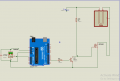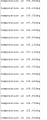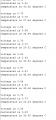# TMP36 reading inaccurate values on arduino

Status
Not open for further replies.

Joined May 11, 2018
131
I am using a TMP36 and arduino (like shown in the connection below ) to measure temperature, and my temperature sensor is changing values abruptly (like from 25deg to 36 deg and back to 25 deg in just a matter of 1sec ).
It looks like there is an error . Can you please tell me if this is normal or not ?

But just for the information i soldered cables on The pins of the TMP36 , i don't know if it affects any how the reading
Thanks
Code:
int sensorPin = 0;
void setup()
{
Serial.begin(9600);
}

void loop()

{
float voltage = reading * 5.0;
float calculated = voltage /= 1024.0;
float temperature = (voltage - 0.5) * 100 ;

Serial.print("voltage is "); Serial.println(voltage);
Serial.print("calculated is "); Serial.println(calculated);
Serial.print("temperature is "); Serial.print(temperature) ;Serial.println(" degrees C");
Serial.println("");

delay(1000);
}the output looks like thisLast edited:

#### crutschow

Joined Mar 14, 2008
25,274
Is the TMP36 pin 3 ground connected directly to the Arduino GND pin?

Joined May 11, 2018
131
Yeah it is connectected to the arduino ground

#### djsfantasi

Joined Apr 11, 2010
6,724
Can you post your sketch (using code tags)?

Joined May 11, 2018
131

Joined May 11, 2018
131

#### djsfantasi

Joined Apr 11, 2010
6,724
Your map statement magnifies the changes reported by the sensor. All the calculations result in a small change reported by the TMP36 looking like a small change.

When reading analog values, it is best to report/calculate based on the original value if possible. Or to use a rolling average for the result.

Just for education, also print out the original value read as well as the calculated temperature.

Joined May 11, 2018
131
Your map statement magnifies the changes reported by the sensor. All the calculations result in a small change reported by the TMP36 looking like a small change.

When reading analog values, it is best to report/calculate based on the original value if possible. Or to use a rolling average for the result.

Just for education, also print out the original value read as well as the calculated temperature.
Thanks
I did change iti think the problem is in the read because it reads a different value every time

Joined May 11, 2018
131
Thanks
I did change it
View attachment 211479
Code:
int sensorPin = 0;
void setup()
{
Serial.begin(9600);
}

void loop()

{
float voltage = reading * 5.0;
float calculated = voltage /= 1024.0;
float temperature = (voltage - 0.5) * 100 ;

Serial.print("voltage is "); Serial.println(voltage);
Serial.print("calculated is "); Serial.println(calculated);
Serial.print("temperature is "); Serial.print(temperature) ;Serial.println(" degrees C");
Serial.println("");

delay(1000);
}
i think the problem is in the read because it reads a different value every time

Last edited:

#### Chris65536

Joined Nov 11, 2019
208
Is there a capacitor on the AREF pin?

Joined Jan 15, 2015
5,623
With your current setup you may want to try this code sample:
Code:
//TMP36 Pin Variables
int sensorPin = 0; //the analog pin the TMP36's Vout (sense) pin is connected to
//the resolution is 10 mV / degree centigrade with a
//500 mV offset to allow for negative temperatures

/*
* setup() - this function runs once when you turn your Arduino on
* We initialize the serial connection with the computer
*/
void setup()
{
Serial.begin(9600);  //Start the serial connection with the computer
//to view the result open the serial monitor
}

void loop()                     // run over and over again
{
//getting the voltage reading from the temperature sensor

// converting that reading to voltage, for 3.3v arduino use 3.3
float voltage = reading * 5.0;
voltage /= 1024.0;

// print out the voltage
Serial.print(voltage); Serial.println(" volts");

// now print out the temperature
float temperatureC = (voltage - 0.5) * 100 ;  //converting from 10 mv per degree wit 500 mV offset
//to degrees ((voltage - 500mV) times 100)
Serial.print(temperatureC); Serial.println(" degrees C");

// now convert to Fahrenheit
float temperatureF = (temperatureC * 9.0 / 5.0) + 32.0;
Serial.print(temperatureF); Serial.println(" degrees F");

delay(1000);                                     //waiting a second
}
When posting code it's also nice to include remarks in your code making it easier for people to follow. The above TMP 36 code sample came from here. You may want to read that link and see how the accuracy of the measurement plane can be improved. Notice in the above code how the offset is handled.

Ron

Joined May 11, 2018
131
Is there a capacitor on the AREF pin?
no there is no capacitor at the Aref

Joined May 11, 2018
131
With your current setup you may want to try this code sample:
Code:
//TMP36 Pin Variables
int sensorPin = 0; //the analog pin the TMP36's Vout (sense) pin is connected to
//the resolution is 10 mV / degree centigrade with a
//500 mV offset to allow for negative temperatures

/*
* setup() - this function runs once when you turn your Arduino on
* We initialize the serial connection with the computer
*/
void setup()
{
Serial.begin(9600);  //Start the serial connection with the computer
//to view the result open the serial monitor
}

void loop()                     // run over and over again
{
//getting the voltage reading from the temperature sensor

// converting that reading to voltage, for 3.3v arduino use 3.3
float voltage = reading * 5.0;
voltage /= 1024.0;

// print out the voltage
Serial.print(voltage); Serial.println(" volts");

// now print out the temperature
float temperatureC = (voltage - 0.5) * 100 ;  //converting from 10 mv per degree wit 500 mV offset
//to degrees ((voltage - 500mV) times 100)
Serial.print(temperatureC); Serial.println(" degrees C");

// now convert to Fahrenheit
float temperatureF = (temperatureC * 9.0 / 5.0) + 32.0;
Serial.print(temperatureF); Serial.println(" degrees F");

delay(1000);                                     //waiting a second
}
When posting code it's also nice to include remarks in your code making it easier for people to follow. The above TMP 36 code sample came from here. You may want to read that link and see how the accuracy of the measurement plane can be improved. Notice in the above code how the offset is handled.

Ron
Thanks but i used this one too . it does not work . the problem is in the reading values as you can see from the results . Voltage changes every second and it causes a huge change of temp

#### crutschow

Joined Mar 14, 2008
25,274
Can you look at the signal with an oscilloscope to see how much noise is on the signal?

Can you do a running average of the signal to reduce the variations?

#### andrewmm

Joined Feb 25, 2011
538
So you have 100 mV jumps on the voltage,
that sounds to me like either interference or a bad power / ground connection to the senser.
How long are the wires to the sensor ?

How oftn do you need a reading ?
the numbers seem to be jumping around a centre value, so if you can not make a better power, then a running or exponential average is the answer to remove the glitches.

#### crutschow

Joined Mar 14, 2008
25,274
You could try a small coax cable (such as used for audio) from the sensor to the Arduino.

Joined May 11, 2018
131
Can you look at the signal with an oscilloscope to see how much noise is on the signal?

Can you do a running average of the signal to reduce the variations?
Where should i put the pins at the oscilloscope to find the noise signals . that would be a great idea..

#### djsfantasi

Joined Apr 11, 2010
6,724
I’m not familiar with other micros, but I think you have a problem with the pin numbering on the Arduino.

A value of 0 for sensorPin is not the first analog pin. On the Arduino, you need to use the symbol A0 instead. A different pin symbol is used for the actual pin when used as a digital pin, D14. Pin 0 on an Arduino is RX, received data. Since I guess that pin isn’t used, it’s likely to return random values.

In the beginning of your program, change setting the sensor pin to this:

sensorPin = A0;

...and try again.

•andrewmm

#### MisterBill2

Joined Jan 23, 2018
6,785
In the circuit shown in post #1 there is no collector resistor for Q1, the NPN driver transistor. So whenever it switches on there will be a lot of current flowing in the 12 volts common line. That is quite likely to cause all kinds of problems. OR the actual circuit may not be the same as what we are shown in post #1. No matter what, switching a shorted circuit across the supply will cause unintended results. That is certain.
I see a comment that there are jumps in the voltage. What I described will certainly cause jumps.

Status
Not open for further replies.